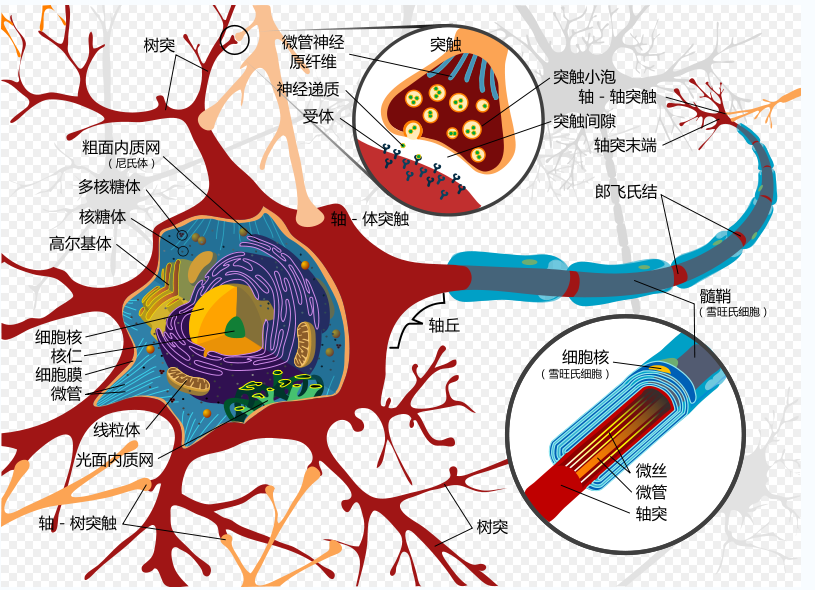# 感知器神经网络

### 感知器（神经网络模型）

感知器是人工神经网络中的一种典型结构， 它的主要的特点是结构简单，对所能解决的问题 存在着收敛算法，并能从数学上严格证明，从而对神经网络研究起了重要的推动作用。神经细胞的状态取决于从其它的神经细胞收到的输入信号量，及突触的强度（抑制或加强）。当信号量总和超过了某个阈值时，细胞体就会激动，产生电脉冲。电脉冲沿着轴突并通过突触传递到其它神经元。为了模拟神经细胞行为，与之对应的感知机基础概念被提出，如权量（突触）、偏置（阈值）及激活函数（细胞体）。 在人工神经网络领域中，感知器也被指为单层的人工神经网络，以区别于较复杂的多层感知器（Multilayer Perceptron）。 作为一种线性分类器，（单层）感知器可说是最简单的前向人工神经网络形式。尽管结构简单，感知器能够学习并解决相当复杂的问题。感知器主要的本质缺陷是它不能处理线性不可分问题。

### 模型构建的代码实现（Python）

import numpy as np
#sigmoid 激活函数
def nonlin(x, deriv = False):
if(deriv==True):
return x*(1-x)
return 1/(1+np.exp(-x))

#输入-输出
#[0,0,1]-0
#[0,1,1]-1
#[1,0,1]-1
#[1,1,1]-0

#输入矩阵
X=np.array([[0,0,1],
[0,1,1],
[1,0,1],
[1,1,1]])

# 目标矩阵
y=np.array([[0,1,0,1]]).T

#生成随机权重
np.random.seed()
weight = np.random.random((3,1))

#训练次数
times=input("How many times you want to train:\n")
times=int(times)

#训练开始
for iter in range(times):
#计算输出
L0=X
L1=nonlin(np.dot(L0,weight))
#计算误差
L1_error=y-L1
L1_delta = L1_error*nonlin(L1,True)
#更新权重

weight+=np.dot(L0.T,L1_delta)

print("Output After Training:")
print(L1)
print("The Weight After Training:")
print(weight)


How many times you want to train:
10000
Output After Training:
[[0.00966633]
[0.99359071]
[0.00786352]
[0.99211866]]
The Weight After Training:
[[-0.20823225]
. [ 9.67307298]
. [-4.6294448 ]]